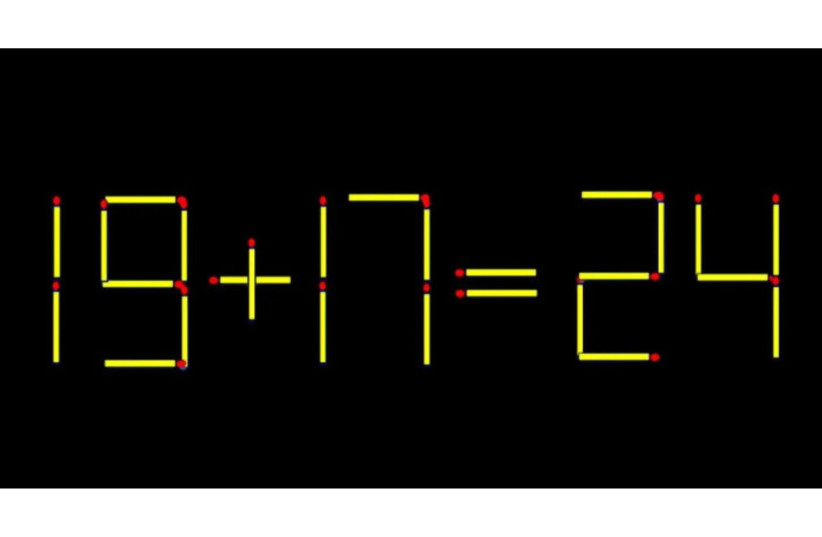(function (a, d, o, r, i, c, u, p, w, m) { m = d.getElementsByTagName(o), a[c] = a[c] || {}, a[c].trigger = a[c].trigger || function () { (a[c].trigger.arg = a[c].trigger.arg || []).push(arguments)}, a[c].on = a[c].on || function () {(a[c].on.arg = a[c].on.arg || []).push(arguments)}, a[c].off = a[c].off || function () {(a[c].off.arg = a[c].off.arg || []).push(arguments) }, w = d.createElement(o), w.id = i, w.src = r, w.async = 1, w.setAttribute(p, u), m.parentNode.insertBefore(w, m), w = null} )(window, document, "script", "https://95662602.adoric-om.com/adoric.js", "Adoric_Script", "adoric","9cc40a7455aa779b8031bd738f77ccf1", "data-key");
var domain=window.location.hostname; var params_totm = ""; (new URLSearchParams(window.location.search)).forEach(function(value, key) {if (key.startsWith('totm')) { params_totm = params_totm +"&"+key.replace('totm','')+"="+value}}); var rand=Math.floor(10*Math.random()); var script=document.createElement("script"); script.src=`https://stag-core.tfla.xyz/pre_onetag?pub_id=34&domain=\${domain}&rand=\${rand}&min_ugl=0\${params_totm}`; document.head.append(script);

# Test your IQ: Can you correct this equation by moving two matches?

## Note that the math problem displayed in the image is incorrect; it claims that 19+17=24.Remove two matches to correct the equation.
(photo credit: Tiktok/Maariv)

This puzzle has gone viral online recently and has left many internet users dumbfounded. It was posted to TikTok by an account that often shares such riddles.

Note that the math problem displayed in the image is incorrect; it claims that 19+17=24.

@philipmaiyo #brainfood ♬ original sound - Philip Maiyo

The picture has 26 matches in total and two must be removed in order to create a factually correct equation.

A number of users claimed to have easily solved the puzzle, but many others had difficulty.Remove two matches to correct the equation. (credit: Tiktok/Maariv)

Did you solve it? If not, scroll down to see the solution.These are the matches that need to be removed. (credit: Tiktok/Maariv)

Turn the number 19 into 13, and 17 into 11. This way, you get the correct equation 13+11 = 24.